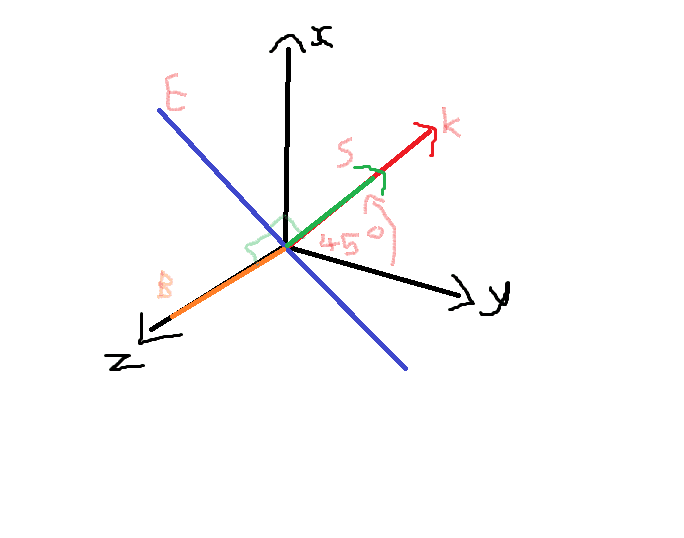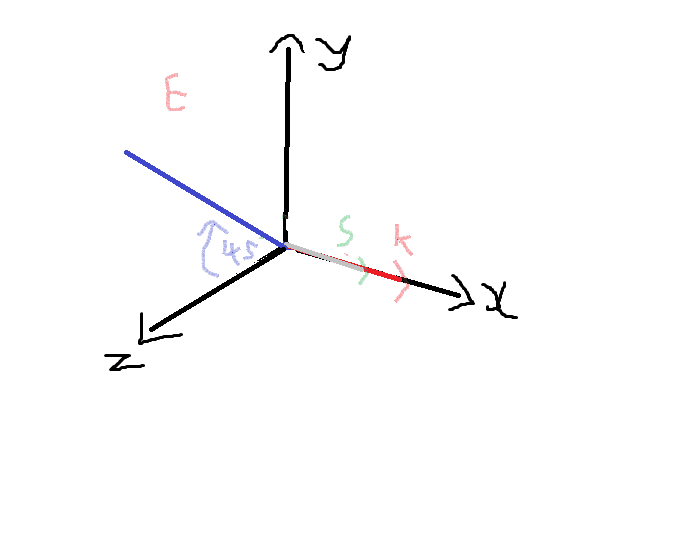# Electromagnetic Waves

## Homework Statement

A plane harmonic electromagnetic wave of amplitude Eo and frequency wo is traveling in the positive x direction in a vacuum. It is linearly polarized with its plane of vibration at 45 degrees to the xy plane and the electric field is zero when x=0 and t=0

a) Make a sketch showing the polarization and propagation direction of the wave. Include the directions of E,B and the Poynting vector in the sketch

b) Write an expression for the electric field of the wave

c) Write an expression for the magnetic field of the wave

## The Attempt at a Solution

a)b) kx = kcos(45), ky = ksin(45), phase angle = cos(x) = 0, therefore x must equal pi/2

E = EoCos(sqrt(2)/2*kx +sqrt(2)/2*ky - wot + pi/2)

c) B = Eo/c*Cos(sqrt(2)/2*kx +sqrt(2)/2*ky - wot + pi/2)

Have I done this correctly and is my sketch correct ?

## Answers and Replies

mfb
Mentor
3D diagrams are always tricky, but the direction of S looks odd and the direction of k is wrong.
What does "travels in x-direction" tell you about k and possible polarization axes?

Why do E and B depend on y?

3D diagrams are always tricky, but the direction of S looks odd and the direction of k is wrong.
What does "travels in x-direction" tell you about k and possible polarization axes?

Why do E and B depend on y?I'm not sure where the magnetic field points. Must it always be perpendicular to the E field and direction of propagation ?

mfb
Mentor
Looks better. You could draw the angle between E and y to make the direction of E clearer.
Must it always be perpendicular to the E field and direction of propagation ?
Right.

rude man
Homework Helper
Gold Member
b) kx = kcos(45), ky = ksin(45), phase angle = cos(x) = 0, therefore x must equal pi/2

E = EoCos(sqrt(2)/2*kx +sqrt(2)/2*ky - wot + pi/2)

c) B = Eo/c*Cos(sqrt(2)/2*kx +sqrt(2)/2*ky - wot + pi/2)

Have I done this correctly and is my sketch correct ?
I'm a bit mystified by your answer to (b) and (c).
E is a vector with y and z components, so is B. Neither has an x component.
I don't see an expression for the vector fields of E or B that I can understand.
Depending on the direction of rotation of the E-B plane, you need to come up with something like
E = Ey j + Ez k
B
= By j - Bz k
including an expression showing the dependency of the E and B vectors with x and t.
where j and k are unit vectors in the y and z direction resp.
(Don't confuse k with k).
Note the + and - signs above. They get reversed if the rotation is in the other direction.